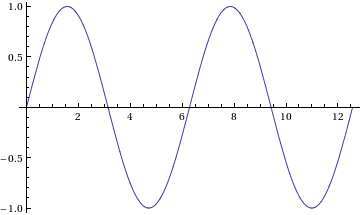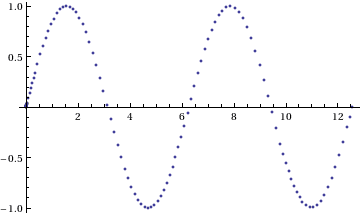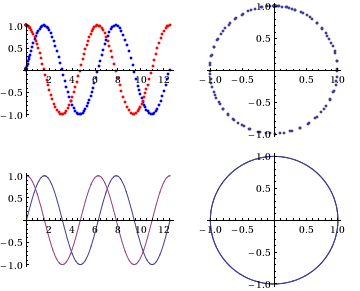# Mathematica - output format

• Mathematica
Hi,
I solved a differential equation using Mathematica using NDsolve, I get some solution, but I
only know how to plot it in Mathematica. The problem is that I need the data - numbers -
and I want to work with then a finally make the graph using gnuplot. Is there any way how
can I cat data in text format?

Export["*.txt", f[t] /. sol, "Table"] but the output was in useless format - I need two columns from
which a could make my own chart . . .

Thaks a lot and sorry for my english.

OK, let's use NDSolve to generate Sin[t] for t from 0 to 4 Pi

Code:
nd = First@NDSolve[x''[t] + x[t] == 0 && x == 0 && x' == 1, x, {t, 0, 4 Pi}]
sin = x /. nd[];
which creates an InterpolatingFunction, which can be plotted to give

Plot[sin[t], {t, 0, 4 Pi}]Now, one way to get a table of data for the solution is to just generated it using Table:
table = Table[sin[t], {t, 0, 4 Pi, .2}]
but this doesn't give the raw data generated by NDSolve.

If you look at the structure of InterpolatingFunction by running, e.g., InputForm[sin], then you see that the t values which NDSolve actually calculated are at the position {3,1}, so can be extracted with sin[[3, 1]]. You can plot their values using

ListPlot[{#, sin[#]} & /@ sin[[3, 1]]]Another way to get at the raw data is to use Sow/Reap to extract it as it is generated:

Code:
{nd, data1} = Reap[NDSolve[(x^\[Prime]'')[t] + x[t] == 0 && x == 0 && x' == 1, x, {t, 0, 4 Pi}, StepMonitor :> Sow[{t, x[t]}]]];
Note that the StepMonitor does not catch the initial {0,0} point, so the full raw data is
Code:
data = Prepend[data1[], {0., 0.}];
We can check that this is the same as the data extracted from the InterpolationFunction since the following will return True:
Code:
data == ({#, sin[#]} & /@ sin[[3, 1]])

Finally, to export the data to a file in your home directory as a pair of columns, try something like

Code:
Export[FileNameJoin[{$HomeDirectory, "sin.txt"}], data, "TSV"] Note that Mathematica tries to guess the format you want from the file extension. ".txt" will just export something like InputForm. In the above I forced the use of tab separated values, but you can also use CSV or Microsoft's XLS, etc... You can see the file produced by either opening it in a text editor or by using Mathematica to print its raw form: Code: FilePrint[FileNameJoin[{$HomeDirectory, "sin.txt"}]]
(* Returns:
0.	0.
0.00010232500915059042	0.00010232500807920588
0.00020465001830118084	0.00020465001401564266
0.003789692138280695	0.0037896791870416077
etc... *)

Note that if you want more points, then it's probably best to use the NDSolve options such as MaxStepSize to generate them. However, you can also just use Table on the original interpolation function to generate the data at any points that you want. Etc...

#### Attachments

Thank you very much! It is working and its great, awsome!

I was trying to google something like that all saturday -
could you just tell where you read it or where can I get these knowlege?

Pretty much everything is in the Mathematica documentation. You just have to piece it all together. As for where I learned it? Well, I've been using Mma for quite a while now, so I guess these are just things you pick up...

Hi, I have just one last problem to this topic - is it possible with the same way
get data for parametric plot? I have system of diffrencial equations and with your help
I cat get f1(x), and f2(x), but I need also table of f1, f2 and manipulating with so many
values is difficult . .. .(I don't understand the principle of mathematica language, so any of
my tries for it doesn't work . .)
thank you.

OK then,

So let's use the same DE as last time, but turn it into a first order one by introducing y[t] == x'[t].
We can numerically integrate it using the optional Reap/Sow/StepMonitor construction
Code:
{nd, data1} = Reap[NDSolve[
y[t] == x'[t] && y'[t] + x[t] == 0 && x == 0 && y == 1,
{x, y}, {t, 0, 4 Pi}, StepMonitor :> Sow[{t, x[t], y[t]}]]];

As before, the full raw data can be defined using
data = Prepend[data1[], {0, 0, 1}];
and the sin = x and cos = y (interpolation) functions can be extracted using
{sin, cos} = {x, y} /. nd[]
We can check that data is the same as what is hidden in the InterpolationFunctions of sin and cos by checking that the following returns True
data == ({#, sin[#], cos[#]} & /@ sin[[3, 1]])

We can then make the plots using the raw data and the interpolation functions
Code:
GraphicsGrid[{
{Show[ListPlot[data[[All, {1, 2}]], PlotStyle -> Blue],
ListPlot[data[[All, {1, 3}]], PlotStyle -> Red]],
ListPlot[data[[All, {2, 3}]], AspectRatio -> 1]},
{Plot[{sin[t], cos[t]}, {t, 0, 4 Pi}],
ParametricPlot[{sin[t], cos[t]}, {t, 0, 4 Pi}]}}]I hope that this is useful... if you need further details on any step, just ask.
But first, I suggest you try to break down the various constructions to see how they work.
Make small changes to test your understanding, etc...